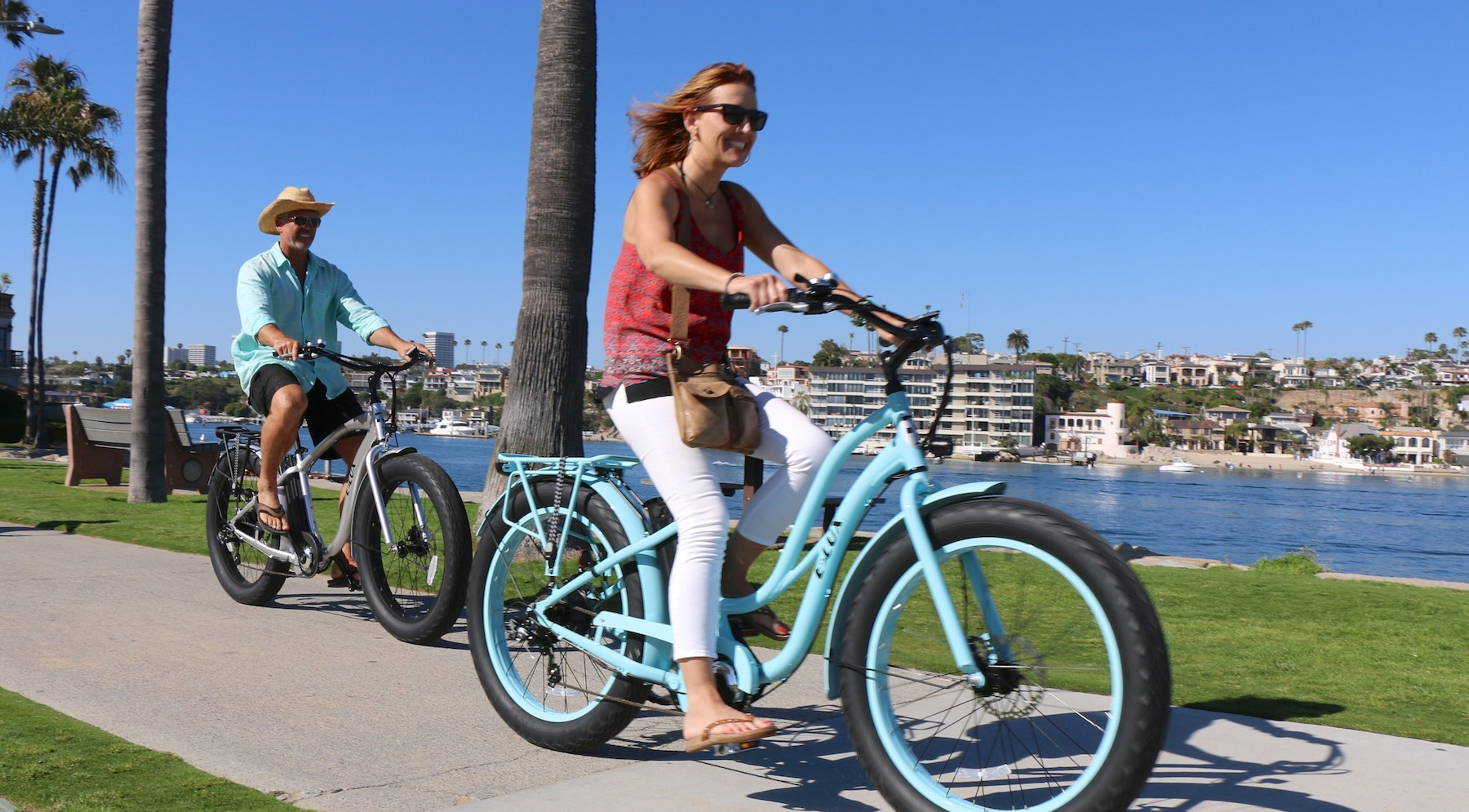If you know at least a few things about electric bikes or even just electricity, you have probably heard the terms volt, watt and amp before. You likely know that they are units of measurement. However, you may not know exactly what they mean. Furthermore, you may be unsure of how they will impact your electric bike performance.

## Volts, Watts and Amps

Before exploring the impact of these units of measurement on electric bike performance, it is important to understand what they measure. They are defined in the International System of Units. Amps (short for amperes) are a base unit, and watts and volts are derived from amps.

• Amp: Amperage is the strength of a current of electricity. Specifically, it is the electromagnetic force between electrical conductors.
• Watt: Wattage is a measurement of power. In other words, it describes the rate of energy transfer.
• Volt: Voltage is the potential for energy to move through a system. It is often compared to water pressure creating the potential for water flow.
• These three measurements are closely related. A watt is equal to one amp of current flowing through an electrical potential of one volt. In other words, the power of a system is equal to the current times the energy contained in the system.

## How They Apply to Electric Bikes

When you hear these terms related to electric bikes, they describe the different characteristics of motors and batteries in the electric powertrain. Wattage describes the power that the powertrain is able to put out. Typically, the peak wattage of the motor is used. So, in very simple terms, the wattage is how powerful the electric bike is (there are some caveats to this, described below).

Voltage is typically used to describe the power that the battery can put out. Batteries typically have a voltage that is a multiple of 12. So, the voltage may be 12, 24, 36 or 48, for example. A lot of volts mean that a battery can deliver a lot of power to the motor. However, it is possible to have excess voltage that is going unused.

Bikes do not typically have anything measured in amps because it is not a useful way to measure current in the real world. Instead, they use ampere-hours or amps multiplied by time. One ampere-hour means that the system can supply a current of one amp for an hour (or two amps for half an hour etc.). Ampere-hours are used to describe the capacity of a battery.

Finding the right electric bike is all about balancing these three factors. High wattage means that the bike can deliver lots of power (and get to higher speeds). However, that will likely be using up the battery power quickly. So, you will need a relatively high ampere-hour battery to compensate. Many electric bikes use 48-volt batteries. However, this can vary. You don’t want to have low voltage constraining your battery output.